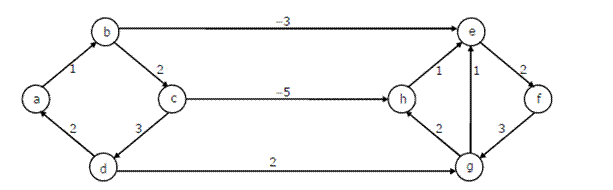# Data Structures and Algorithms | Set 15

• Difficulty Level : Medium
• Last Updated : 27 Mar, 2017

Following questions have been asked in GATE CS 2008 exam.

1. The most efficient algorithm for finding the number of connected components in an undirected graph on n vertices and m edges has time complexity.

(A) Θ(n)
(B) Θ(m)
(C) Θ(m + n)
(D) Θ(mn)

Connected components can be found in O(m + n) using Tarjan’s algorithm. Once we have connected components, we can count them.

2. Consider the Quicksort algorithm. Suppose there is a procedure for finding a pivot element which splits the list into two sub-lists each of which contains at least one-fifth of the elements. Let T(n) be the number of comparisons required to sort n elements. Then

(A) T(n) <= 2T(n/5) + n (B) T(n) <= T(n/5) + T(4n/5) + n (C) T(n) <= 2T(4n/5) + n (D) T(n) <= 2T(n/2) + n Answer (B) For the case where n/5 elements are in one subset, T(n/5) comparisons are needed for the first subset with n/5 elements, T(4n/5) is for the rest 4n/5 elements, and n is for finding the pivot. If there are more than n/5 elements in one set then other set will have less than 4n/5 elements and time complexity will be less than T(n/5) + T(4n/5) + n because recursion tree will be more balanced.
3 Dijkstra’s single source shortest path algorithm when run from vertex a in the below graph, computes the correct shortest path distance to(A) only vertex a
(B) only vertices a, e, f, g, h
(C) only vertices a, b, c, d
(D) all the vertices

Dijkstra’s single source shortest path is not guaranteed to work for graphs with negative weight edges, but it works for the given graph.
Let us see…

Let us run the 1st pass
b 1
b is minimum, so shortest distance to b is 1.

After 1st pass, distances are
c 3, e -2.
e is minimum, so shortest distance to e is -2

After 2nd pass, distances are
c 3, f 0.
f is minimum, so shortest distance to f is 0

After 3rd pass, distances are
c 3, g 3.
Both are same, let us take g. so shortest distance to g is 3.

After 4th pass, distances are
c 3, h 5
c is minimum, so shortest distance to c is 3

After 5th pass, distances are
h -2
h is minimum, so shortest distance to h is -2

4. The following C function takes a single-linked list of integers as a parameter and rearranges the elements of the list. The function is called with the list containing the integers 1, 2, 3, 4, 5, 6, 7 in the given order. What will be the contents of the list after the function completes execution?

 `struct` `node ``{``  ``int` `value;``  ``struct` `node *next;``};``void` `rearrange(``struct` `node *list)``{``  ``struct` `node *p, * q;``  ``int` `temp;``  ``if` `((!list) || !list->next) ``      ``return``;``  ``p = list;``  ``q = list->next;``  ``while``(q) ``  ``{``     ``temp = p->value;``     ``p->value = q->value;``     ``q->value = temp;``     ``p = q->next;``     ``q = p?p->next:0;``  ``}``}`

(A) 1,2,3,4,5,6,7
(B) 2,1,4,3,6,5,7
(C) 1,3,2,5,4,7,6
(D) 2,3,4,5,6,7,1